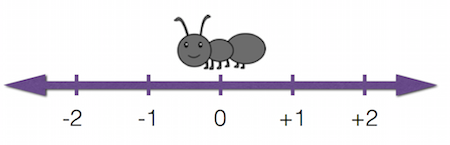Andy the Ant is on a number line at the location 0. Each minute, he travels 1 unit to the right with probability $\frac{3}{4}$ and 1 unit to the left with probability $\frac{1}{4}$. What is the expected value for Andy’s location after one hour?